# Post Order Traversal of Binary Tree in O(N) using O(1) space

Prerequisites:- Morris Inorder Traversal, Tree Traversals (Inorder, Preorder and Postorder)
Given a Binary Tree, the task is to print the elements in post order using O(N) time complexity and constant space.

```Input:   1
/   \
2       3
/ \     / \
4   5   6   7
/ \
8   9
Output: 4 8 9 5 2 6 7 3 1

Input:   5
/   \
7       3
/ \     / \
4   11  13  9
/ \
8   4
Output: 4 8 4 11 7 13 9 3 5
```

Method 1: Using Morris Inorder Traversal

1. Create a dummy node and make the root as it’s left child.
2. Initialize current with dummy node.
3. While current is not NULL
• If current does not have a left chil traverse the right child, current = current->right
• Otherwise,
1. Find the right most child in the left subtree.
2. If right most child’s right child is NULL
• Make current as the right child of the right most node.
• Traverse the left child, current = current->left
3. Otherwise,
• Set the right most child’s right pointer to NULL.
• From current’s left child, traverse along the right children until the right most child and reverse the pointers.
• Traverse back from right most child to current’s left child node by reversing the pointers and printing the elements.
4. Traverse the right child, current = current->right

Below is the diagram showing the right most child in left subtree, pointing to it’s inorder successor.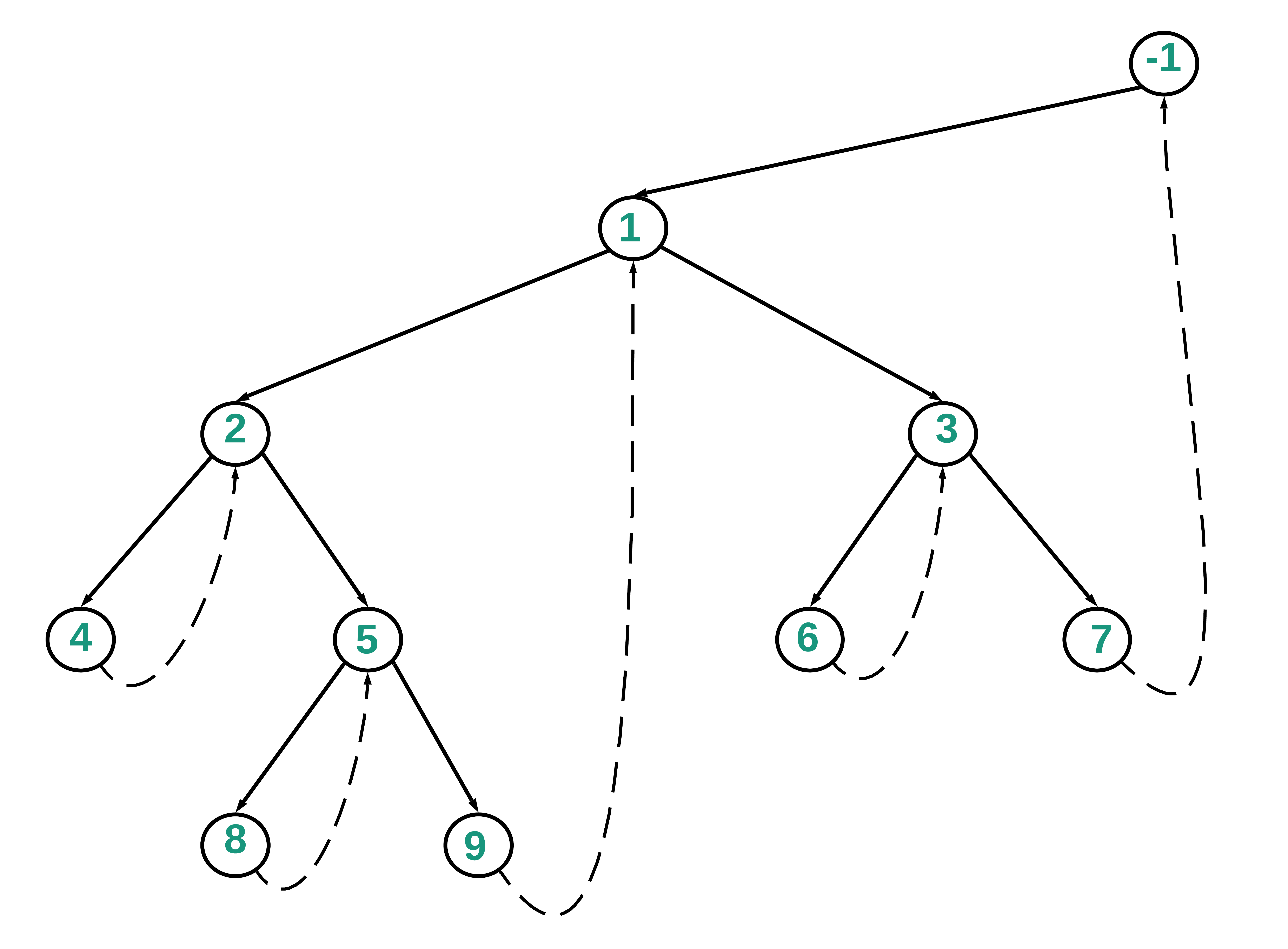Below is the diagram which highlights the path 1->2->5->9 and the way the nodes are processed and printed as per the above algorithm.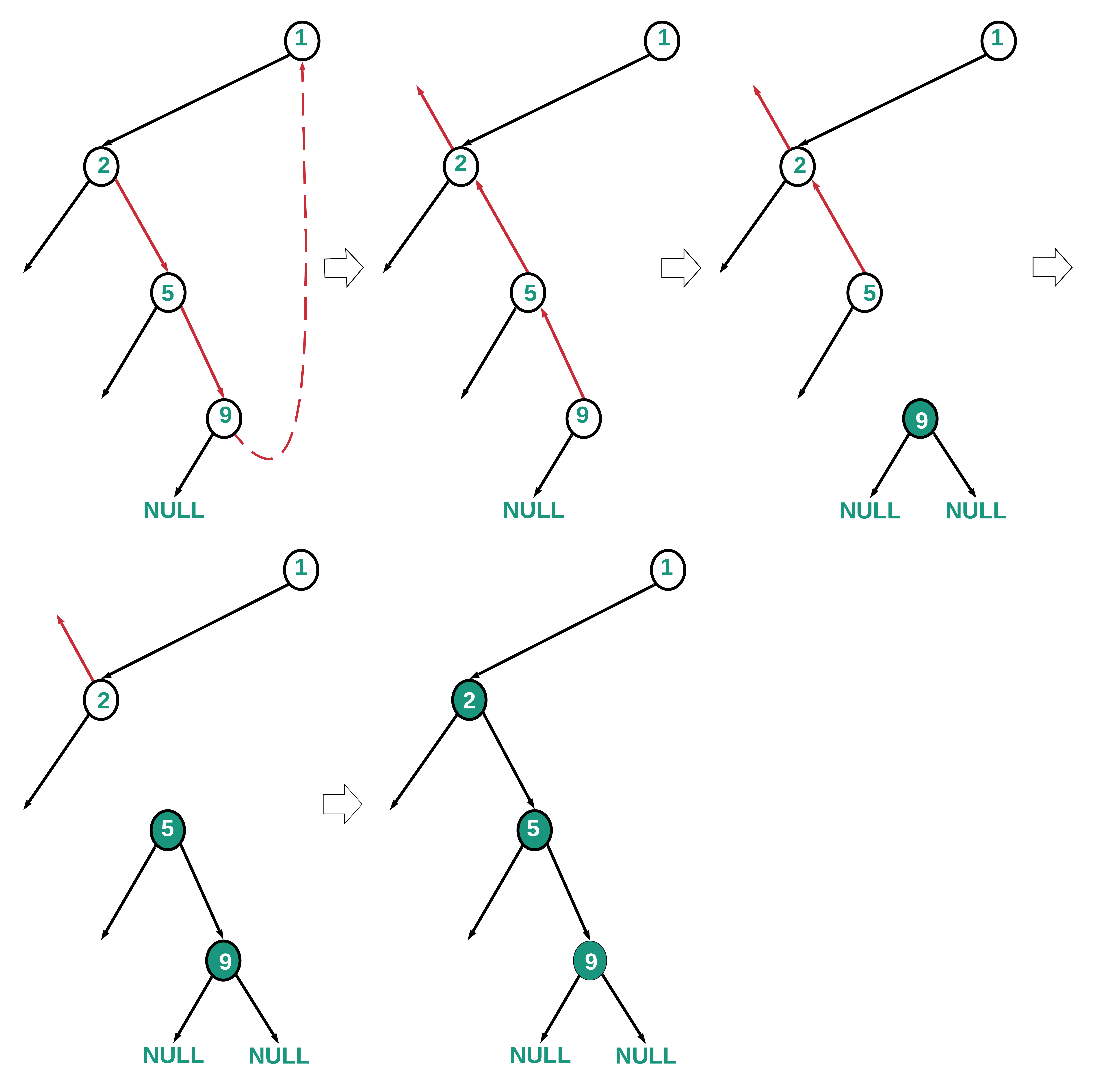Below is the implementation of the above approach:

## C++

 `// C++ program to implement  ` `// Post Order traversal  ` `// of Binary Tree in O(N)  ` `// time and O(1) space  ` `#include   ` `using` `namespace` `std;  ` ` `  `class` `node  ` `{  ` `    ``public``:  ` `    ``int` `data;  ` `    ``node *left, *right;  ` `};  ` ` `  `// Helper function that allocates a  ` `// new node with the given data and  ` `// NULL left and right pointers.  ` `node* newNode(``int` `data)  ` `{  ` `    ``node* temp = ``new` `node();  ` `    ``temp->data = data;  ` `    ``temp->left = temp->right = NULL;  ` `    ``return` `temp;  ` `}  ` ` `  `// Postorder traversal without recursion ` `// and without stack  ` `void` `postOrderConstSpace(node* root)  ` `{  ` `    ``if` `(root == NULL)  ` `            ``return``;  ` ` `  `        ``node* current = newNode(-1);  ` `        ``node* pre = NULL;  ` `        ``node* prev = NULL;  ` `        ``node* succ = NULL;  ` `        ``node* temp = NULL;  ` `         `  `        ``current->left = root;  ` `         `  `    ``while` `(current)  ` `    ``{  ` `         `  `        ``// If left child is null.  ` `        ``// Move to right child.  ` `        ``if` `(current->left == NULL)  ` `        ``{  ` `            ``current = current->right;  ` `        ``}  ` `        ``else` `        ``{  ` `            ``pre = current->left; ` `             `  `            ``// Inorder predecessor ` `            ``while` `(pre->right &&  ` `                   ``pre->right != current)  ` `                ``pre = pre->right;  ` `             `  `            ``// The connection between current and  ` `            ``// predecessor is made ` `            ``if` `(pre->right == NULL)  ` `            ``{  ` `                 `  `                ``// Make current as the right  ` `                ``// child of the right most node  ` `                ``pre->right = current;  ` `                 `  `                ``// Traverse the left child  ` `                ``current = current->left;  ` `            ``} ` `            ``else` `            ``{  ` `                ``pre->right = NULL;  ` `                ``succ = current;  ` `                ``current = current->left;  ` `                ``prev = NULL;  ` `                 `  `                ``// Traverse along the right  ` `                ``// subtree to the  ` `                ``// right-most child  ` `                ``while` `(current != NULL) ` `                ``{  ` `                    ``temp = current->right;  ` `                    ``current->right = prev;  ` `                    ``prev = current;  ` `                    ``current = temp;  ` `                ``}  ` `                 `  `                ``// Traverse back  ` `                ``// to current's left child ` `                ``// node ` `                ``while` `(prev != NULL) ` `                ``{  ` `                    ``cout << prev->data << ``" "``;  ` `                    ``temp = prev->right;  ` `                    ``prev->right = current;  ` `                    ``current = prev;  ` `                    ``prev = temp;  ` `                ``}  ` ` `  `                ``current = succ;  ` `                ``current = current->right;  ` `            ``}  ` `        ``}  ` `    ``}  ` `}  ` ` `  `// Driver code ` `int` `main()  ` `{  ` `    ``/* Constructed tree is as follows:-  ` `                          ``1   ` `                       ``/     \  ` `                      ``2       3  ` `                     ``/ \     / \  ` `                    ``4   5   6   7  ` `                       ``/ \  ` `                      ``8   9  ` `          ``*/` `    ``node* root = NULL;  ` ` `  `    ``root = newNode(1);  ` `    ``root->left = newNode(2);  ` `    ``root->right = newNode(3);  ` ` `  `    ``root->left->left = newNode(4);  ` `    ``root->left->right = newNode(5);  ` ` `  `    ``root->right->left = newNode(6);  ` `    ``root->right->right = newNode(7);  ` ` `  `    ``root->left->right->left = newNode(8);  ` `    ``root->left->right->right = newNode(9); ` `     `  `    ``postOrderConstSpace(root);  ` `    ``return` `0;  ` `}  ` ` `  `// This code is contributed by Saurav Chaudhary `

## Java

 `// Java program to implement ` `// Post Order traversal ` `// of Binary Tree in O(N) ` `// time and O(1) space ` ` `  `// Definition of the ` `// binary tree ` `class` `TreeNode { ` `    ``public` `int` `data; ` `    ``public` `TreeNode left; ` `    ``public` `TreeNode right; ` `    ``public` `TreeNode(``int` `data) ` `    ``{ ` `        ``this``.data = data; ` `    ``} ` ` `  `    ``public` `String toString() ` `    ``{ ` `        ``return` `data + ``" "``; ` `    ``} ` `} ` ` `  `public` `class` `PostOrder { ` ` `  `    ``TreeNode root; ` ` `  `    ``// Function to find Post Order ` `    ``// Traversal Using Constant space ` `    ``void` `postOrderConstantspace(TreeNode ` `                                    ``root) ` `    ``{ ` `        ``if` `(root == ``null``) ` `            ``return``; ` ` `  `        ``TreeNode current ` `            ``= ``new` `TreeNode(-``1``), ` `            ``pre = ``null``; ` `        ``TreeNode prev = ``null``, ` `                 ``succ = ``null``, ` `                 ``temp = ``null``; ` `        ``current.left = root; ` ` `  `        ``while` `(current != ``null``) { ` ` `  `            ``// Go to the right child ` `            ``// if current does not ` `            ``// have a left child ` ` `  `            ``if` `(current.left == ``null``) { ` `                ``current = current.right; ` `            ``} ` ` `  `            ``else` `{ ` ` `  `                ``// Traverse left child ` `                ``pre = current.left; ` ` `  `                ``// Find the right most child ` `                ``// in the left subtree ` `                ``while` `(pre.right != ``null` `                       ``&& pre.right != current) ` `                    ``pre = pre.right; ` ` `  `                ``if` `(pre.right == ``null``) { ` ` `  `                    ``// Make current as the right ` `                    ``// child of the right most node ` `                    ``pre.right = current; ` ` `  `                    ``// Traverse the left child ` `                    ``current = current.left; ` `                ``} ` ` `  `                ``else` `{ ` `                    ``pre.right = ``null``; ` `                    ``succ = current; ` `                    ``current = current.left; ` `                    ``prev = ``null``; ` ` `  `                    ``// Traverse along the right ` `                    ``// subtree to the ` `                    ``// right-most child ` ` `  `                    ``while` `(current != ``null``) { ` `                        ``temp = current.right; ` `                        ``current.right = prev; ` `                        ``prev = current; ` `                        ``current = temp; ` `                    ``} ` ` `  `                    ``// Traverse back from ` `                    ``// right most child to ` `                    ``// current's left child node ` ` `  `                    ``while` `(prev != ``null``) { ` ` `  `                        ``System.out.print(prev); ` `                        ``temp = prev.right; ` `                        ``prev.right = current; ` `                        ``current = prev; ` `                        ``prev = temp; ` `                    ``} ` ` `  `                    ``current = succ; ` `                    ``current = current.right; ` `                ``} ` `            ``} ` `        ``} ` `    ``} ` ` `  `    ``// Driver Code ` `    ``public` `static` `void` `main(String[] args) ` `    ``{ ` `        ``/* Constructed tree is as follows:- ` `                          ``1  ` `                       ``/     \ ` `                      ``2       3 ` `                     ``/ \     / \ ` `                    ``4   5   6   7 ` `                       ``/ \ ` `                      ``8   9 ` `          ``*/` `        ``PostOrder tree = ``new` `PostOrder(); ` `        ``tree.root = ``new` `TreeNode(``1``); ` `        ``tree.root.left = ``new` `TreeNode(``2``); ` `        ``tree.root.right = ``new` `TreeNode(``3``); ` `        ``tree.root.left.left = ``new` `TreeNode(``4``); ` `        ``tree.root.left.right ` `            ``= ``new` `TreeNode(``5``); ` `        ``tree.root.right.left ` `            ``= ``new` `TreeNode(``6``); ` `        ``tree.root.right.right ` `            ``= ``new` `TreeNode(``7``); ` `        ``tree.root.left.right.left ` `            ``= ``new` `TreeNode(``8``); ` `        ``tree.root.left.right.right ` `            ``= ``new` `TreeNode(``9``); ` ` `  `        ``tree.postOrderConstantspace( ` `            ``tree.root); ` `    ``} ` `} `

Output

`4 8 9 5 2 6 7 3 1 `

Time Complexity: O(N)
Auxiliary Space: O(1)
Method 2: In method 1, we traverse a path, reverse references, print nodes as we restore the references by reversing them again. In method 2, instead of reversing paths and restoring the structure, we traverse to parent node from the current node using the current node’s left subtree. This could be faster depending on the tree structure, for example in a right-skewed tree.
The following algorithm and diagrams provide the details of the approach.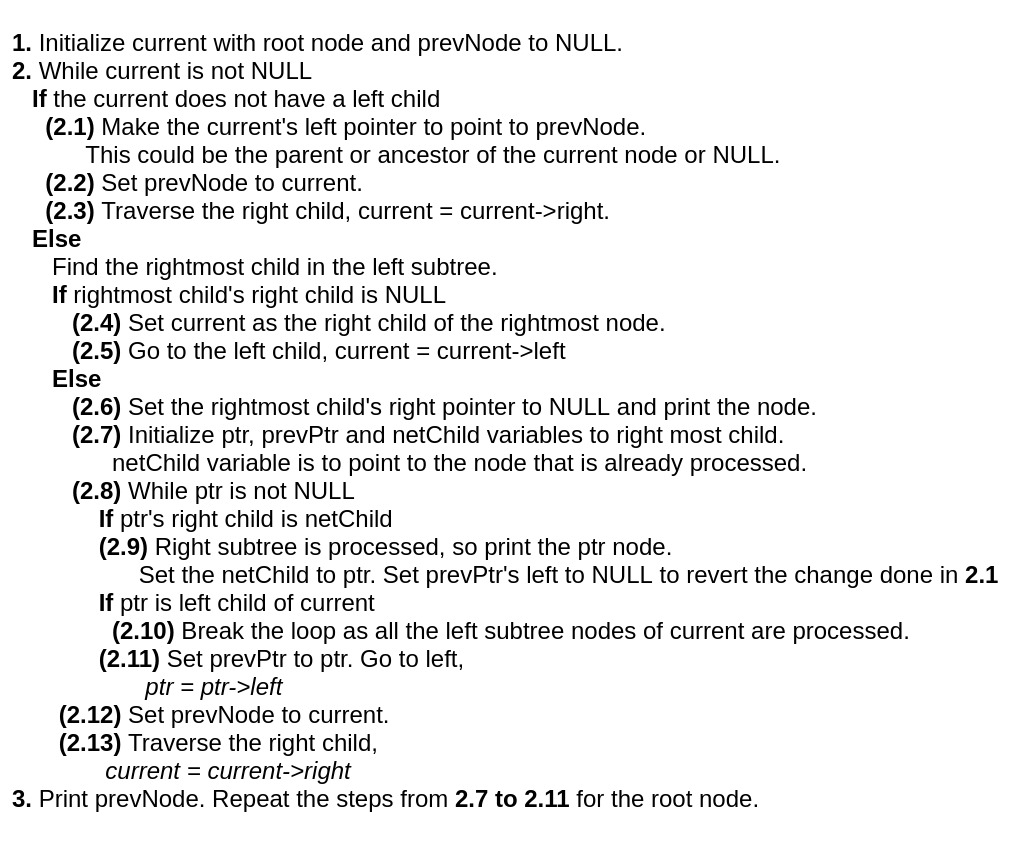Below is the conceptual diagram showing how the left and right child references are used to traverse back and forth.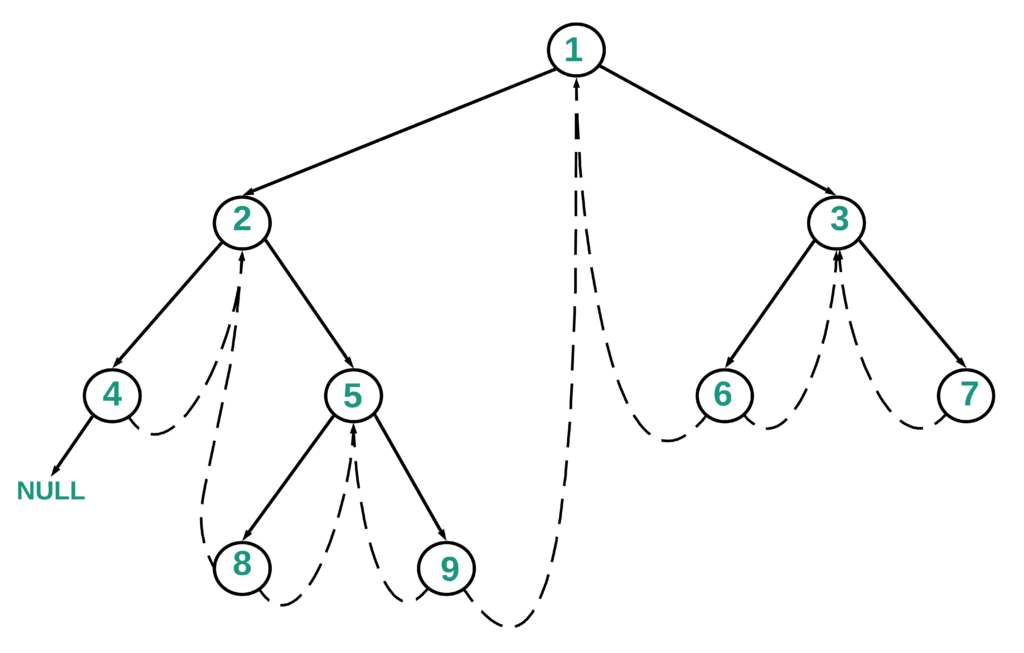Below is the diagram which highlights the path 1->2->5->9 and the way the nodes are processed and printed as per the above algorithm.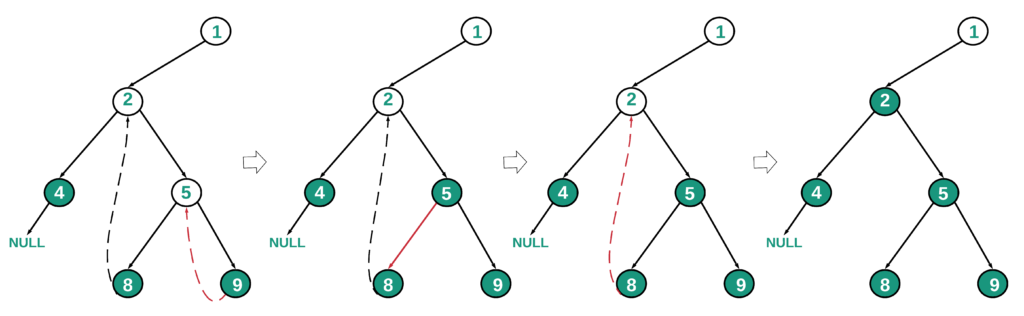Below is the implementation of the above approach:

## Java

 `// Java Program to implement ` `// the above approach ` `class` `TreeNode { ` `    ``public` `int` `data; ` `    ``public` `TreeNode left; ` `    ``public` `TreeNode right; ` ` `  `    ``public` `TreeNode(``int` `data) ` `    ``{ ` `        ``this``.data = data; ` `    ``} ` ` `  `    ``public` `String toString() ` `    ``{ ` `        ``return` `data + ``" "``; ` `    ``} ` `} ` ` `  `public` `class` `PostOrder { ` `    ``TreeNode root; ` ` `  `    ``// Function to Calculate Post ` `    ``// Order Traversal ` `    ``// Using Constant Space ` `    ``void` `postOrderConstantspace(TreeNode root) ` `    ``{ ` `        ``if` `(root == ``null``) ` `            ``return``; ` ` `  `        ``TreeNode current = ``null``; ` `        ``TreeNode prevNode = ``null``; ` `        ``TreeNode pre = ``null``; ` `        ``TreeNode ptr = ``null``; ` `        ``TreeNode netChild = ``null``; ` `        ``TreeNode prevPtr = ``null``; ` ` `  `        ``current = root; ` `        ``while` `(current != ``null``) { ` `            ``if` `(current.left == ``null``) { ` `                ``current.left = prevNode; ` `                ``// Set prevNode to current ` `                ``prevNode = current; ` `                ``current = current.right; ` `            ``} ` `            ``else` `{ ` `                ``pre = current.left; ` `                ``// Find the right most child ` `                ``// in the left subtree ` `                ``while` `(pre.right != ``null` `                       ``&& pre.right != current) ` `                    ``pre = pre.right; ` ` `  `                ``if` `(pre.right == ``null``) { ` `                    ``pre.right = current; ` `                    ``current = current.left; ` `                ``} ` `                ``else` `{ ` `                    ``// Set the right most ` `                    ``// child's right pointer ` `                    ``// to NULL ` `                    ``pre.right = ``null``; ` `                    ``System.out.print(pre); ` ` `  `                    ``ptr = pre; ` `                    ``netChild = pre; ` `                    ``prevPtr = pre; ` `                    ``while` `(ptr != ``null``) { ` `                        ``if` `(ptr.right == netChild) { ` `                            ``System.out.print(ptr); ` `                            ``netChild = ptr; ` `                            ``prevPtr.left = ``null``; ` `                        ``} ` ` `  `                        ``if` `(ptr == current.left) ` `                            ``break``; ` `                        ``// Break the loop ` `                        ``// all the left subtree ` `                        ``// nodes of current ` `                        ``// processed ` ` `  `                        ``prevPtr = ptr; ` `                        ``ptr = ptr.left; ` `                    ``} ` ` `  `                    ``prevNode = current; ` `                    ``current = current.right; ` `                ``} ` `            ``} ` `        ``} ` ` `  `        ``System.out.print(prevNode); ` ` `  `        ``// Last path traversal ` `        ``// that includes the root. ` `        ``ptr = prevNode; ` `        ``netChild = prevNode; ` `        ``prevPtr = prevNode; ` `        ``while` `(ptr != ``null``) { ` `            ``if` `(ptr.right == netChild) { ` `                ``System.out.print(ptr); ` `                ``netChild = ptr; ` `                ``prevPtr.left = ``null``; ` `            ``} ` `            ``if` `(ptr == root) ` `                ``break``; ` ` `  `            ``prevPtr = ptr; ` `            ``ptr = ptr.left; ` `        ``} ` `    ``} ` ` `  `    ``// Main Function ` `    ``public` `static` `void` `main(String[] args) ` `    ``{ ` `        ``/* Constructed tree is as follows:- ` `                          ``1  ` `                       ``/     \ ` `                      ``2       3 ` `                     ``/ \     / \ ` `                    ``4   5   6   7 ` `                       ``/ \ ` `                      ``8   9 ` `          ``*/` `        ``PostOrder tree = ``new` `PostOrder(); ` `        ``tree.root = ``new` `TreeNode(``1``); ` `        ``tree.root.left = ``new` `TreeNode(``2``); ` `        ``tree.root.right = ``new` `TreeNode(``3``); ` `        ``tree.root.left.left ` `            ``= ``new` `TreeNode(``4``); ` `        ``tree.root.left.right ` `            ``= ``new` `TreeNode(``5``); ` `        ``tree.root.right.left ` `            ``= ``new` `TreeNode(``6``); ` `        ``tree.root.right.right ` `            ``= ``new` `TreeNode(``7``); ` `        ``tree.root.left.right.left ` `            ``= ``new` `TreeNode(``8``); ` `        ``tree.root.left.right.right ` `            ``= ``new` `TreeNode(``9``); ` ` `  `        ``tree.postOrderConstantspace( ` `            ``tree.root); ` `    ``} ` `} `

Output:

```4 8 9 5 2 6 7 3 1
```

Time Complexity: O(N)
Auxiliary Space: O(1)

Attention reader! Don’t stop learning now. Get hold of all the important DSA concepts with the DSA Self Paced Course at a student-friendly price and become industry ready.

My Personal Notes arrow_drop_upCheck out this Author's contributed articles.

If you like GeeksforGeeks and would like to contribute, you can also write an article using contribute.geeksforgeeks.org or mail your article to contribute@geeksforgeeks.org. See your article appearing on the GeeksforGeeks main page and help other Geeks.

Please Improve this article if you find anything incorrect by clicking on the "Improve Article" button below.

Improved By : sauravchaudhary717